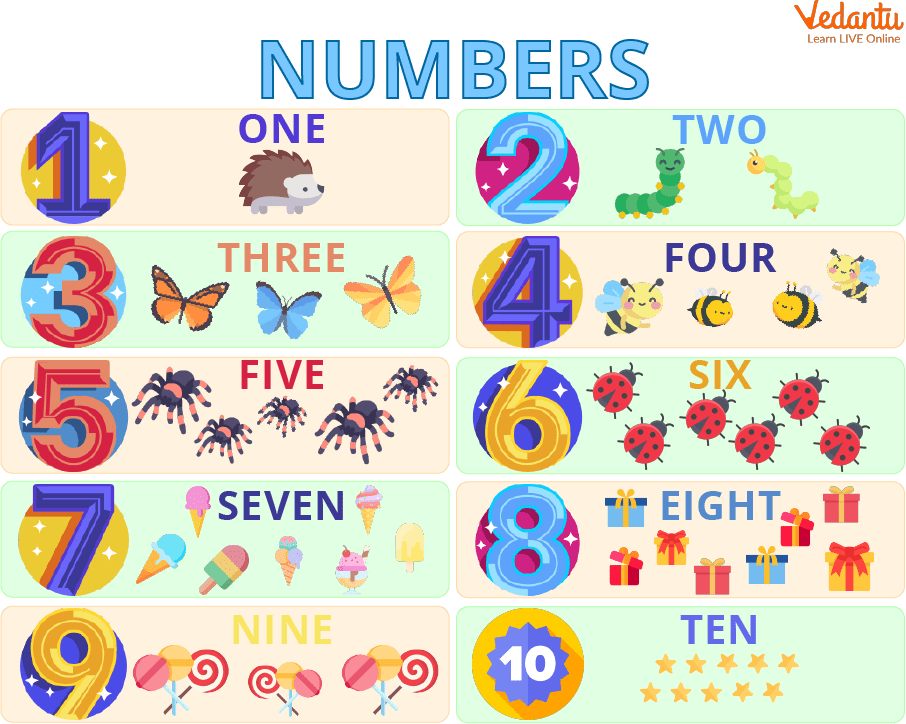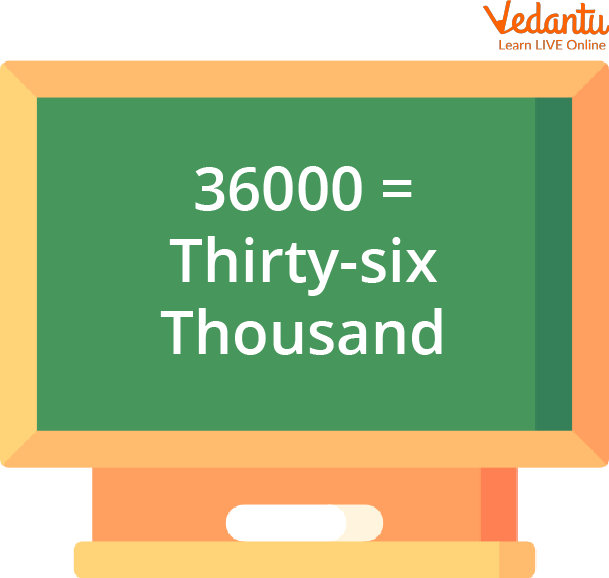Courses
Courses for Kids
Free study material
Free LIVE classes
More

# 33600 in WordsLIVE
Join Vedantu’s FREE Mastercalss

## Introduction to Number Names

In daily life, we encounter many numbers in our life. For buying things, calculating scores, calculating marks in the result, calculation of toffees, all things require number knowledge. Here we have mentioned below how all the numbers are represented in the word format from 1 to 1000.Number Names

Number knowledge is very important to learning maths as numbers serve as the building blocks of maths. With the help of them, kids learn to spell the numbers correctly as well. This maths activity is taught to all the kids in primary school. Writing numbers in words with the help of English letters is very useful in learning maths subjects.

In this article, we will mainly focus on how to write 33600 in words.

Some Basic Numbers in Words

1- one

2- two

3- three

4- four

5- five

6- six

7- seven

8- eight

9- nine

10- ten

## Standard Form

In this method, we write a number simply in digits. This is the basic form of writing a number. This is the initial method for writing number names.

Some examples of numbers in standard form;

1. 20

2. 31

3. 44

4. 25

5. 55

6. 100

7. 231

8. 2456

9. 20954

10. 5555678

## Number in Words or Word Form

Writing numbers in words or numbers names is another form of writing numbers. For writing numbers in words, one should know the spelling of the number.

Some examples of numbers in words;

5- five                                         91-ninety one

13-thirteen                                101-one hundred one

21-twenty one                          103-one hundred three

33- thirty-three                         203- two hundred three

45-forty five                               309-three hundred nine

56-fifty six                                   406-four hundred six

67-sixty seven                            507-five hundred seven

79-seventy nine                         805- eight hundred five

80-eighty                                    907- nine hundred seven

## Expanded Form

In this method, the number is written according to its place value. For writing numbers in expanded form, one should have the knowledge of place values.

Some numbers in expanded form;

1. 5,467 - 5,000+400+60+7

2. 56,789 - 50,000+6000+700+80+9

3. 7,65,564 - 7,00,000+60,000+5000+500+60+7

## How to write Numbers in Words

Here, the steps for writing numbers in words are covered. Any number must first be checked for place values before being written down in words. Let's discover this by following the instructions below.

One, two, three, four, five, six, seven, eight, and nine are the terms used to represent the place value of a number from 1 to 9. The smallest number that is in the tens place is 10, which is represented by the word ten.

## How to Write 36000 in Words

In 36,000, 3 is at ten thousand places, six is at thousands place and 0 at hundreds, tens and ones place.

So 36000 words can be written as “Thirty-Six Thousand”.The Number 36000

• 36000 is an even number.

• It is a composite number

• The expanded form of 36000 is 30,000+6,000+0+0+0

• 36000 is not a perfect square and a cube.

## Different Methods of writing 36000

• In standard form, the above number is written as 36,000.

• In expanded form, it can be written as 30,000+6,000+0+0+0.

• 36000 in words, is written as Thirty Thousand Six Hundred.

## Solved Examples

Q1 Write in words the value of 21 + 41 - (25 + 19).

Ans: Simplifying 21 + 41 - (25 + 19) gives:

⇒ 21 + 41 - (25 + 19) = 21 + 41 - 44 = 62 - 44 = 18

Using the number names chart, 18 is written as:

⇒ 10 + 8 = Ten + Eight = Eighteen

∴ 21 + 41 - (25 + 19) in words = Eighteen.

Q2. What is the Value of Sixty-One Minus Forty-Six?

Ans: In numerals, sixty-one is written as 61 and forty-six is written as 46.

Now, 61 - 46 = 15.

Using the number names chart, 15 is written as:

⇒ 10 + 5 = Ten + Five = Fifteen.

Q3 Find the Value of 6 + 7. Write the Answer in Words.

Ans: On simplifying, 6 + 7 gives 13 and 13 in words is written as:

⇒ 10 + 3 = Ten + Three = Thirteen.

## Practice Questions

Q1. What is 123 in words?

Ans: One hundred twenty-three.

Q2. What is the number name of 48000?

Ans: Forty-Eight Thousand

Q3. What is the standard form of Five Hundred Eighty-Six?

Ans: 586

Q4. What is the expanded form of seven thousand twenty-one?

Ans: 7000+200+20+1

Q5. Write the number name of 555.

Ans: Five-Hundred Fifty Five

## Summary

Standard form, number in words and expanded form are the different methods of writing numbers. 36000 is an even number and composite number. 36000 in words, is written as Thirty Thousand Six Hundred. For a better understanding, similar toys can be used as numbers, and you can play games with your kids by asking them the numbers in words randomly. They will enjoy and learn at the same time. All mathematical operations are based on these numbers, not only in primary classes but in higher classes also. In the above context, we learnt some methods of writing numbers, how to write 36000 words and many other important things.

Last updated date: 03rd Oct 2023
Total views: 123.3k
Views today: 3.23k

## FAQs on 33600 in Words

1. What are the number names?

Words that contain numbers are written with the English alphabet. Number names are a more attractive way of writing numbers for kids. Both words and numbers can be used to express numbers. For example: 1,00,000 in words is written as One Lakh or 1,00,000.

2. On what exactly is the number system based?

The number system is based on the place value of the digits, such as ones, tens, hundreds, thousands, and so forth; any natural numbers can be expressed in words. In 1,00,000, 1 is at a lakh place, and 0 is at ten thousand, thousand, hundred, tens and ones place. The number names given in the article above should be thoroughly memorised by the kids.

3. What is the international number system?

There are two methods of writing numbers. One is the Indian system, and the other is the International number system. In India, both systems are taught to the students. The place value names in the international system differ from the Indian number system. For instance, in the Indian system, 1,000,000 is equal to 10 lakhs, and, in the international system, it is equal to 1 million. In the international number system, the place values of digits go in the sequence of Ones, Tens, Hundreds, Thousands, Ten Thousand, Hundred Thousands, Millions, Ten Million and so on. For teaching this to your child, you can prefer playing some board games with them.